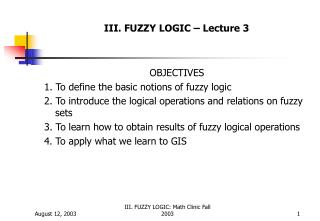DownloadDownload PresentationIII. FUZZY LOGIC – Lecture 3

# III. FUZZY LOGIC – Lecture 3

Télécharger la présentation## III. FUZZY LOGIC – Lecture 3

- - - - - - - - - - - - - - - - - - - - - - - - - - - E N D - - - - - - - - - - - - - - - - - - - - - - - - - - -
##### Presentation Transcript

1. III. FUZZY LOGIC – Lecture 3 OBJECTIVES 1. To define the basic notions of fuzzy logic 2. To introduce the logical operations and relations on fuzzy sets 3. To learn how to obtain results of fuzzy logical operations 4. To apply what we learn to GIS III. FUZZY LOGIC: Math Clinic Fall 2003

2. OUTLINEIII. FUZZY LOGIC A. Introduction B. Inputs to fuzzy logic systems - fuzzification C. Fuzzy propositions D. Fuzzy hedges E. Computing the results of a fuzzy proposition given an input F. The resulting action III. FUZZY LOGIC: Math Clinic Fall 2003

3. A. Introduction (figure from Earl Cox) III. FUZZY LOGIC: Math Clinic Fall 2003

4. Introduction Steps (Earl Cox based on previous slide): 1. Input – vocabulary, fuzzification (creating fuzzy sets) 2. Fuzzy propositions – IF X is Y THEN Z (or Z is A) … there are four types of propositions 3. Hedges – very, extremely, somewhat, more, less 4. Combination and evaluation – computation of the results given the inputs 5. Action - defuzzification III. FUZZY LOGIC: Math Clinic Fall 2003

5. Input – vocabulary, fuzzification (creating a fuzzy set) by using our previous methods of frequency, combination, experts/surveys (figure from Earl Cox) III. FUZZY LOGIC: Math Clinic Fall 2003

6. Input (figure from Klir&Yuan) III. FUZZY LOGIC: Math Clinic Fall 2003

7. Fuzzy Propositions – types 1 and 2 GENERAL FORMS 1. Unconditional and unqualified proposition: Q is P Example: Temperature(Q) is high(P) 2. Unconditional and qualified proposition: proposition(Q is P) is R Example: That Coimbra and Catania are beautiful is very true. III. FUZZY LOGIC: Math Clinic Fall 2003

8. Fuzzy Proposition – type 1 and 2 (from Earl Cox) III. FUZZY LOGIC: Math Clinic Fall 2003

9. Fuzzy Propositions – type 1 and 2 (from Earl Cox) III. FUZZY LOGIC: Math Clinic Fall 2003

10. Fuzzy Propositions – type 3 3. Conditional and unqualified proposition: IF Q is P THEN R is S Example: If Robert is tall, then clothes are large. If car is slow, then gear is low. III. FUZZY LOGIC: Math Clinic Fall 2003

11. Fuzzy Propositions – type 4 4. Conditional and qualified proposition: IF Q is P THEN R is S is T {proposition(IF Q is P THEN R is S )} is T III. FUZZY LOGIC: Math Clinic Fall 2003

12. Fuzzy Hedges (from Earl Cox) III. FUZZY LOGIC: Math Clinic Fall 2003

13. Fuzzy Hedges (from Earl Cox) III. FUZZY LOGIC: Math Clinic Fall 2003

14. Illustrations of Fuzzy Propositions – Composition/Evaluation (from Klir&Yuan) III. FUZZY LOGIC: Math Clinic Fall 2003

15. Illustrations of Fuzzy Propositions – Composition/Evaluation (Earl Cox) III. FUZZY LOGIC: Math Clinic Fall 2003

16. Illustrations of Fuzzy Propositions – Composition/Evaluation (from Earl Cox) III. FUZZY LOGIC: Math Clinic Fall 2003

17. Illustrations of Fuzzy Propositions Decomposition – Defuzzification/Action (from Earl Cox) III. FUZZY LOGIC: Math Clinic Fall 2003

18. Defuzzification (from Earl Cox) III. FUZZY LOGIC: Math Clinic Fall 2003

19. Defuzzification (from Earl Cox) III. FUZZY LOGIC: Math Clinic Fall 2003